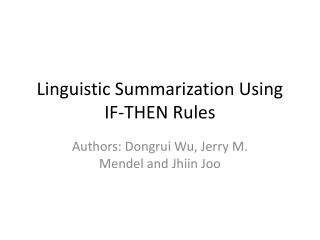DownloadDownload PresentationLinguistic Summarization Using IF-THEN Rules

# Linguistic Summarization Using IF-THEN Rules

Télécharger la présentation## Linguistic Summarization Using IF-THEN Rules

- - - - - - - - - - - - - - - - - - - - - - - - - - - E N D - - - - - - - - - - - - - - - - - - - - - - - - - - -
##### Presentation Transcript

1. Linguistic Summarization Using IF-THEN Rules Authors: Dongrui Wu, Jerry M. Mendel and JhiinJoo

2. Introduction • Type I & Type II Fuzzy Systems • Dataset Description • Linguistic Descriptions • Implementation

3. Type-1 Fuzzy Sets • Crisp sets, where x  A or x  A • Membership is a continuous grade  [0,1] • Membership a value Degree of “Tall-ness”  1 0.6 0 Height (m) 1.77

4. Interval Type-2 Fuzzy Sets • Interval type-2 fuzzy sets - interval membership grades • X is primary domain • Jx is the secondary domain • All secondary grades (A(x,u)) equal 1 • A(x) is the secondary membership function at x (vertical slice representation) ~ A = {((x,u), 1) | x  X, u  Jx, Jx  [0,1]} ~

5. Interval Type-2 Fuzzy Sets • Membership no longer crisp ~ Upper Membership Function Tall  1 Type -1 MF = FOU (explained in next slide) 0 Height (m) Lower MF Tall

6. Interval Type-2 Fuzzy Sets • Fuzzification: ~ Tall  1 0.78 Tall (1.8) = [0.42,0.78] ~ 0.42 0 Height (m) 1.8

7. Interval Type-2 Fuzzy Sets • FOU • Vertical slice of a Type 2 membership function • Indicating 3D structure of Type 2 Mendel Jerry M. and. Bob John Robert I, “Type-2 Fuzzy Sets Made Simple.” IEEE TRANSACTIONS ON FUZZY SYSTEMS, VOL. 10, NO. 2, APRIL 2002.

8. Haberman’s survival Data set - UIUC • From a study conducted between 1958 and 1970 at the University of Chicago's Billings Hospital on the survival of patients who had undergone surgery for breast cancer. • Attribute Information: • 1. Age of patient at time of operation (numerical) 2. Patient's year of operation (year - 1900, numerical) 3. Number of positive axillary nodes detected (numerical) 4. Survival status (class attribute) -- 1 = the patient survived 5 years or longer -- 2 = the patient died within 5 year

9. Linguistic Summarizations: IF - THEN • Type 1: IFAGE is 35 AND YEAR is 1962, THENSURVIVAL is YES • Type 2: IFAGE is around 35 AND YEAR is around1962, THENSURVIVAL is YES

10. Some parameters • T – Degree of Truth; an assessment of Validity • T increases as more data satisfying antecedent also satisfy consequent

11. Some parameters • C – Degree of Sufficient Coverage • Determines if sufficient data satisfies a rule • (trigger) • C=f(rc) • U – Degree of Usefulness • Indicates how useful a rule is • A rule is useful iff • it has high degree of truth: most of the data satisfy the rule’s antecedents as well as its consequent • It has sufficient coverage: enough data are described by it. • U=min(T,C) • It depends on the parameters described earlier

12. Some parameters • O – Degree of Outlier • Indicates if a rule describes the outliers instead of most of the data • If T=0, O=0 since no data is described by the rule • Described by the complement of T & C since they both depend on the data (not outlier)

13. Some parameters • S - Degree of Simplicity • Determined by the length of the summary • L = number of antecedents • Simplest rule: S=1 (one antecedent and one consequent)

14. MAMC Rules • Multi Antecedent Multi consequent

15. Implementation • Each case represented as a piecewise linear curve • Blue – strength of supporting rule • Red- cases violating given rule • Black- Irrelevant • Figure shows if C is used for ranking, T may/may not be high

16. Implementation • Figure shows if U is used for ranking, high U indicates high T & C : useful rule

17. Conclusions • An important method of ranking rules using the parameters: • Degree of Truth • Degree of Sufficient Coverage • Degree of Usefulness • Degree of Outlier • Degree of Simplicity